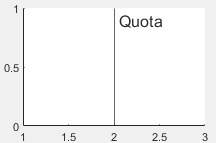# xline

## 语法

xline(x)
xline(x,LineSpec)
xline(x,LineSpec,labels)
xline(___,Name,Value)
xline(ax,___)
xl = xline(___)

## 说明

xline(x) 在当前坐标区的一个或多个 x 坐标处创建一条垂直线。例如，xline(2) 在 x=2 处创建一条线。

xline(x,LineSpec) 指定线型、线条颜色或同时指定两者。例如，xline([12 20 33],'--b') 创建三条蓝色虚线。

xline(x,LineSpec,labels) 为线条添加标签。

xline(___,Name,Value) 使用一个或多个名称-值对组参数指定常量线属性。有关属性列表，请参阅 ConstantLine 属性。请在所有其他输入参数之后指定名称-值对组。

xline(ax,___) 在指定的坐标区（而不是当前坐标区）中创建线条。

xl = xline(___) 返回一个 ConstantLine 对象或 ConstantLine 对象数组。使用 xl 修改或查询所创建的线条的属性。

## 示例

xline(3);x = linspace(0,6,100); y = exp(x); plot(x,y) xline(4.5,'-',{'Acceptable','Limit'});data = 5 * randn(1,500) + 20; histogram(data,'FaceAlpha',0.1,'EdgeAlpha',0.1); m = mean(data); s = std(data); xline([m-s m m+s],'-',{'-1 Standard Dev.','Average','+1 Standard Dev.'})xline(5,'--r');y = [3187 2693 1771 1826 1958 3222 1645]; barh(y,'DisplayName','Daily Sales') xl = xline(2328,'-.','Average','DisplayName','Average Sales');xl.LabelVerticalAlignment = 'middle'; xl.LabelHorizontalAlignment = 'center'; legend('show');tiledlayout(2,1) ax1 =nexttile; x = linspace(0,10,200); y1 = cos(x); plot(ax1,x,y1) ax2 = nexttile; y2 = sin(x); plot(ax2,x,y2) xline(ax1,pi/2,':','cos(\pi/2)=0'); xline(ax2,pi,':','sin(\pi)=0');## 输入参数

x 坐标，指定为标量或包含数值、分类、日期时间或持续时间值的向量。x 的数据类型必须与 x 轴的数据类型匹配。

-实线
--虚线
:点线
-.点划线

'o'圆圈
'+'加号
'*'星号
'.'
'x'叉号
'_'水平线条
'|'垂直线条
's'方形
'd'菱形
'^'上三角
'v'下三角
'>'右三角
'<'左三角
'p'五角形
'h'六角形

y

m

c

r

g

b

w

k

#### 为一条线创建标签

xline(12,'-','Sample')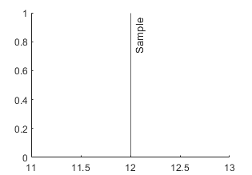xline(12,'-',{'Sample','x=12'})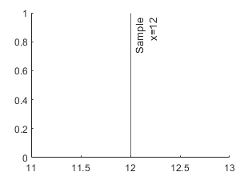#### 为多行创建标签

xline([13 20 33],'-','Sample')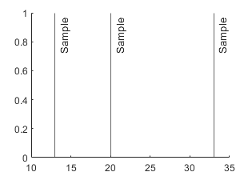label = {'Sample 1','Sample 2','Sample 3'}; xline([13 20 33],'-',label)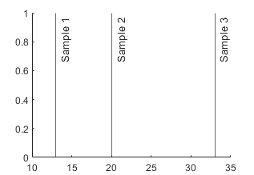label = {'Sample 1',sprintf('Sample 2\nx=20'),'Sample 3'}; xline([13 20 33],'-',label)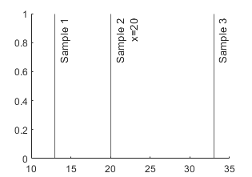### 名称-值对组参数

• RGB 三元组是包含三个元素的行向量，其元素分别指定颜色中红、绿、蓝分量的强度。强度值必须位于 [0,1] 范围内，例如 [0.4 0.6 0.7]

• 十六进制颜色代码是字符向量或字符串标量，以井号 (#) 开头，后跟三个或六个十六进制数字，范围可以是 0F。这些值不区分大小写。因此，颜色代码 '#FF8800''#ff8800''#F80''#f80' 是等效的。

'red''r'[1 0 0]'#FF0000''green''g'[0 1 0]'#00FF00''blue''b'[0 0 1]'#0000FF''cyan' 'c'[0 1 1]'#00FFFF''magenta''m'[1 0 1]'#FF00FF''yellow''y'[1 1 0]'#FFFF00''black''k'[0 0 0]'#000000''white''w'[1 1 1]'#FFFFFF''none'不适用不适用不适用无颜色

RGB 三元组十六进制颜色代码外观
[0 0.4470 0.7410]'#0072BD'[0.8500 0.3250 0.0980]'#D95319'[0.9290 0.6940 0.1250]'#EDB120'[0.4940 0.1840 0.5560]'#7E2F8E'[0.4660 0.6740 0.1880]'#77AC30'[0.3010 0.7450 0.9330]'#4DBEEE'[0.6350 0.0780 0.1840]'#A2142F''right'线的右侧。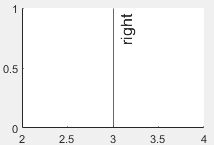'left'线的左侧。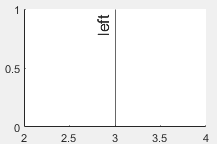'center'线的中心。标签将线分段。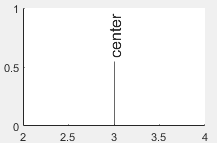'top'线的顶部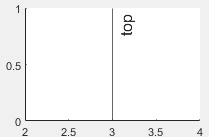'middle'线的中间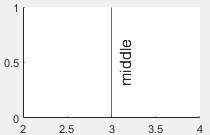'bottom'线的底部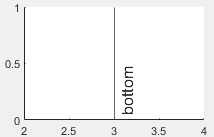'aligned'标签与线的方向相同。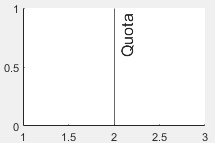'horizontal'无论线的方向如何，标签始终为水平方向。